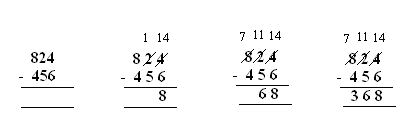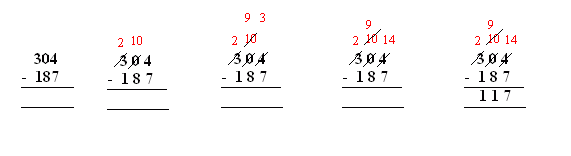Name: ___________________Date:___________________

 Email us to get an instant 20% discount on highly effective K-12 Math & English kwizNET Programs!

Grade 3 - Mathematics5.14 Subtraction - 3 digit

 Subtraction without regrouping:Start subtracting from the ones place. If the subtrahend is lesser than minuend in ones place then we borrow 1 from tens place. If the subtrahend is lesser than minuend in tens place then we borrow 1 from hundreds place Answer: 533 Subtraction with regrouping:Start from the ones place, 4 is lesser than 6. So 1 is borrowed from 2 and 4 becomes 14. In tens place, 2 becomes 1, now again 1 is less than 5, so 1 is borrowed from 8 and 1 becomes 11. In ones place 4 is subtracted from 7. Answer: 368 Subtracting across zeros:When there is a zero it is always made 10 by borrrowing 1 from digits in higher place values. In ones place 4 is made 14 and zero is made 9 (since 1 is borrowed from 10 to make 14) In hundreds place 3 becomes 2. Answer: 117 Directions: Subtract the following. Also write at least ten examples of your own.
 Q 1: 485 - 123 =462361362 Q 2: 328 - 129 =201200199 Q 3: 500 - 250 =250350450 Q 4: 921 - 721 =201102200 Q 5: 300 -158 =252142152 Q 6: 100 - 95 =550105 Q 7: 682 - 190 =512592492 Q 8: 711 - 699 =12120188 Question 9: This question is available to subscribers only! Question 10: This question is available to subscribers only!